1.1(2).2

In [Ko01] p.46, the homogeneous pair (g,h) with index 1.1(2).2 is defined as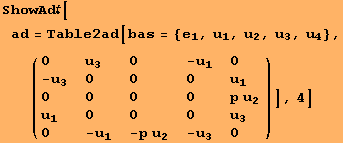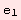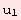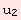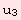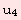00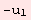00 0 00 0 0 0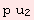0 0 00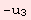0

for p∈R. Any ρ-invariant scalarproduct B is of the form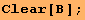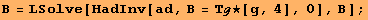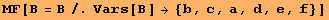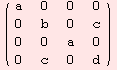The tensor ν:g×gm is determined by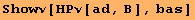00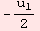0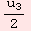0 00 0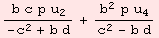0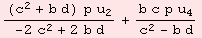0 0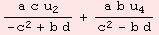0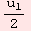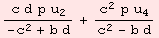The Levi-Civita connection Λ:ggl(m) is determined by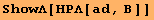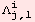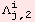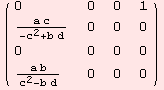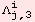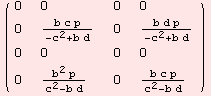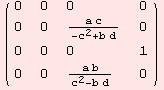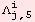The non-zero evaluations of the Riemannian-curvature tensor R:m×mgl(m) are determined by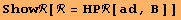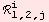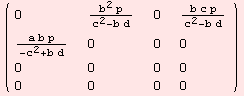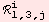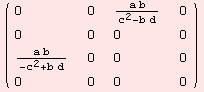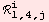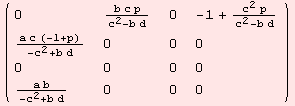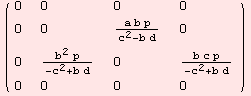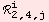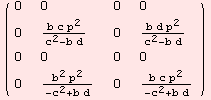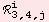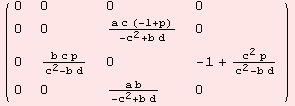The Ricci-curvature Ric:m×mR is determined by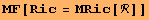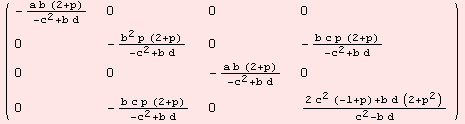We demand Ric=0. Thus, b=0 and p=1.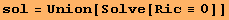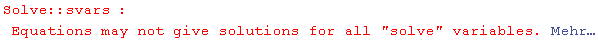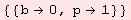But then, the Riemannian curvature tensor vanishes also.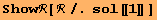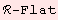Note, this result is independent of the signature of B.

 Created by Mathematica  (August 15, 2006)